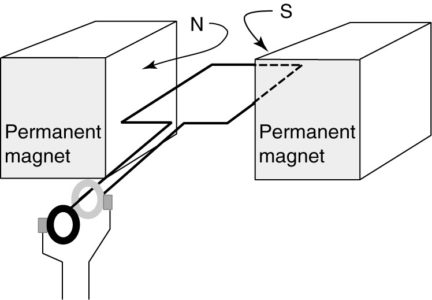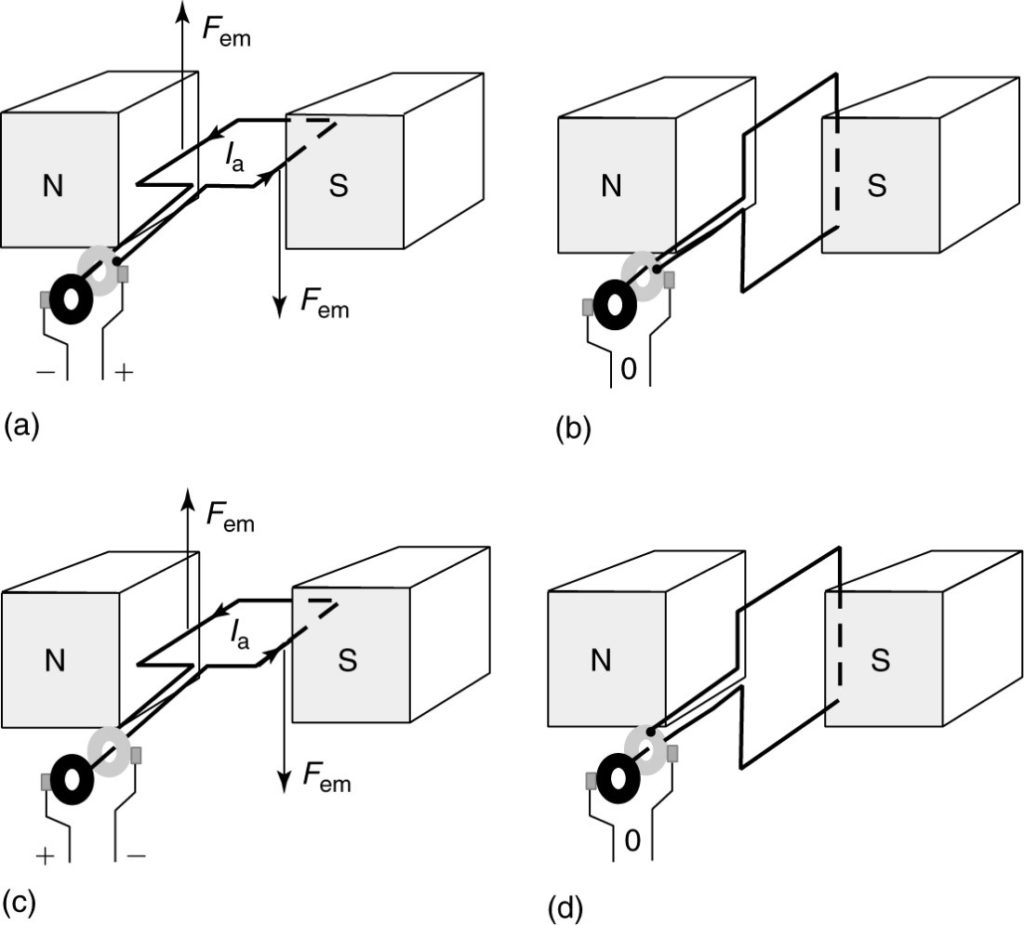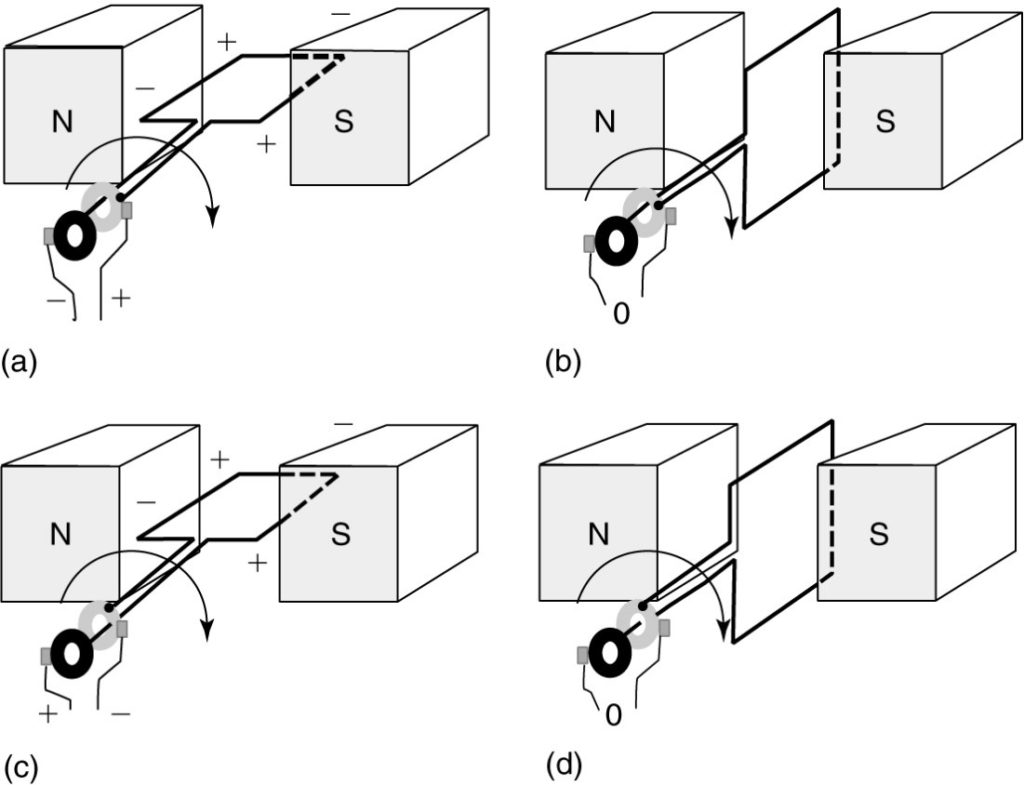Home / Synchronous Machines / AC Motor and Generator Working Principle

# AC Motor and Generator Working Principle

Want create site? Find Free WordPress Themes and plugins.

Figure 1 shows a simple, single-turn, permanent- magnet machine. The single-turn coil is free to rotate between two magnetic poles of opposite polarity. Magnetic flux goes from a north pole to a south pole, so there will be magnetic flux in the region between the poles where the coil is rotating. Obviously, there would have to be some type of support to hold the coil in place as it is rotating.

The assembly that rotates is called the rotor of the machine, and the stationary poles would be called the stator. For this particular geometry, the rotor delivers power and is also called the armature, and the stator provides a magnetic field and is thus called the field.FIGURE 1    A simple permanent-magnet AC machine.

In order to connect the coil to a source or load, we must make connections that still allow the coil to rotate. Such a connection is provided by a slip ring.

Slip rings are conducting rings placed around, but insulated from, the shaft of the rotor. The dark-colored slip ring is connected to one end of the coil, while the light-colored slip ring is connected to the other end.

Conducting blocks of carbon or carbon and copper, called brushes, are placed against the slip rings to make the connection to the outside of the machine. Thus, the rotating coil either receives (motor operation) or delivers (generator operation) AC electric power via the brushes and slip rings. This simple machine can be operated as a motor or generator.

## AC Motor Operation

Figure 2 shows several views of the machine operating as a motor. An AC voltage is applied to the coil such that the polarity is as shown in Figure 2(a).

In particular, the coil side on the right (next to the South Pole) is connected to the positive terminal. Thus, current flows around the coil as shown.

Since there is a current flowing in the presence of a magnetic field, there will be a force on the conductors. The direction of the force could be found by flux bunching or by the left-hand rule. In any event, the force is down on the right side of the coil and up on the left side, creating a torque that causes the coil to rotate.FIGURE    2 Operation of the simple AC machine as a motor.

As the coil rotates to the position shown in Figure 2(b), the AC voltage is assumed to pass through zero.

Note that even if the voltage were applied in this position, no torque would be created because the force on the top part of the coil would be up and that on the bottom part of the coil would be down.

Assuming there is some inertia in the rotor to keep it moving through the neutral position, the coils would continue moving to the position shown in Figure 2(c).

The two coil sides have switched positions as compared to 2(a), so the polarity of the applied voltage must change to keep the rotor moving in the same direction. As the coil continues to rotate, it reaches the neutral position shown in Figure 2(d), which is the same as 2(b) except that the coil has rotated 180°.

One important thing to notice from the operation of this simple motor is that the rotation speed must be synchronized to the frequency of the applied voltage. This type of motor is called a synchronous motor, although as we will see the construction of a practical synchronous motor is somewhat different than shown here.

## AC Generator Operation

Consider what happens as the coil is rotated in the direction shown in Figure 3.

In Figure 3(a), both coil sides are adjacent to magnetic poles, so they are cutting flux, which induces a voltage in each side of the coil. In particular, the side of the coil connected to the darker slip ring is adjacent to the North Pole.

Using the right-hand generator rule, the voltage induced in the coil is found to have the polarity shown, causing the darker slip ring to have negative polarity.

The other coil side, connected to the light-colored slip ring is under the South Pole and produces a voltage that adds to the voltage of the other side, causing the light-colored slip ring to have a positive polarity.FIGURE 3    Operation of the simple AC machine as a generator.

As the coil sides rotate, they sweep out a circular path. The velocity of each coil side at any instant in time is tangent to the circular path or perpendicular to the plane of the coil. Thus, the voltage generated by the coil is not constant.

In particular, consider Figure 3(b). Here, the conductor’s velocity is parallel to the flux and no voltage is generated. As the coil continues to rotate, the conductor connected to the dark slip ring comes under the South Pole, so the dark ring becomes the positive terminal. In other words, this is an AC generator.

Figures 3(c) and (d) show the rest of one cycle. Note that one revolution delivers one cycle of voltage, so the frequency of the voltage would be equal to the number of revolutions per second. If the machine was driven at a constant speed, the frequency would also be constant. Thus, we call this machine a synchronous generator.

The voltage generated by this machine would essentially be sinusoidal, and from Faraday’s law we can derive the same expression we found for the voltage in a transformer coil (equation 1), except that we use the flux per pole instead of the maximum flux:

$\begin{matrix} {{E}_{rms}}=4.44fN{{\phi }_{p}} & {} & \left( 1 \right) \\\end{matrix}$

Obviously, not every machine is designed to run at the same speed. It is desirable to use different types of prime movers, such as steam turbines, diesel engines, or water wheels to drive synchronous generators.

Each prime mover has its own operating speed range, so we need to be able to build generators with different operating speeds. Fortunately, with proper design of the generator, this can be done within certain limits.

Figure 4 shows another simple generator, but this time there are four magnetic poles, two north, and two south, arranged in alternating fashion. The only part of the rotor coil is shown, for clarity.

As we have seen, when one side of the coil is under a north pole and one is under the South Pole, the voltages induced in the two sides add. Thus, the coil shown in Figure 4 has one side under the North Pole and one under the South Pole.

There would be a second coil adjacent to the other two poles, and the two rotor coils could be operated in parallel or series, depending upon the design of the machine.

The coil physically spans 90°; however, we consider a north-south pole pair to be 180°, electrically speaking. This leads to the concept of electrical and mechanical degrees:

$\begin{matrix} {{\theta }_{elec}}=\frac{P}{2}\times {{\theta }_{mech}} & {} & \left( 2 \right) \\\end{matrix}$

Where P is the number of poles in the machine (always an even number).FIGURE 4    A four-pole AC machine.

When the coil in Figure 4 is driven by a prime mover, it generates two complete cycles of voltage while it makes one revolution. Thus, it would have to go only half as fast as the two-pole machine to operate at the same frequency. In order to compute the synchronous speed in RPM, we use the following formula:

$\begin{matrix} {{n}_{s}}=\frac{60\times f}{\frac{P}{2}}RPM & {} & \left( 3 \right) \\\end{matrix}$

Or

$\begin{matrix} {{n}_{s}}=\frac{120\times f}{P}RPM & {} & \left( 3 \right) \\\end{matrix}$

Where ns is the synchronous speed of the machine in RPM and f is the frequency of the AC voltage. Equation 3 is a very important equation for AC machines.

Did you find apk for android? You can find new Free Android Games and apps.